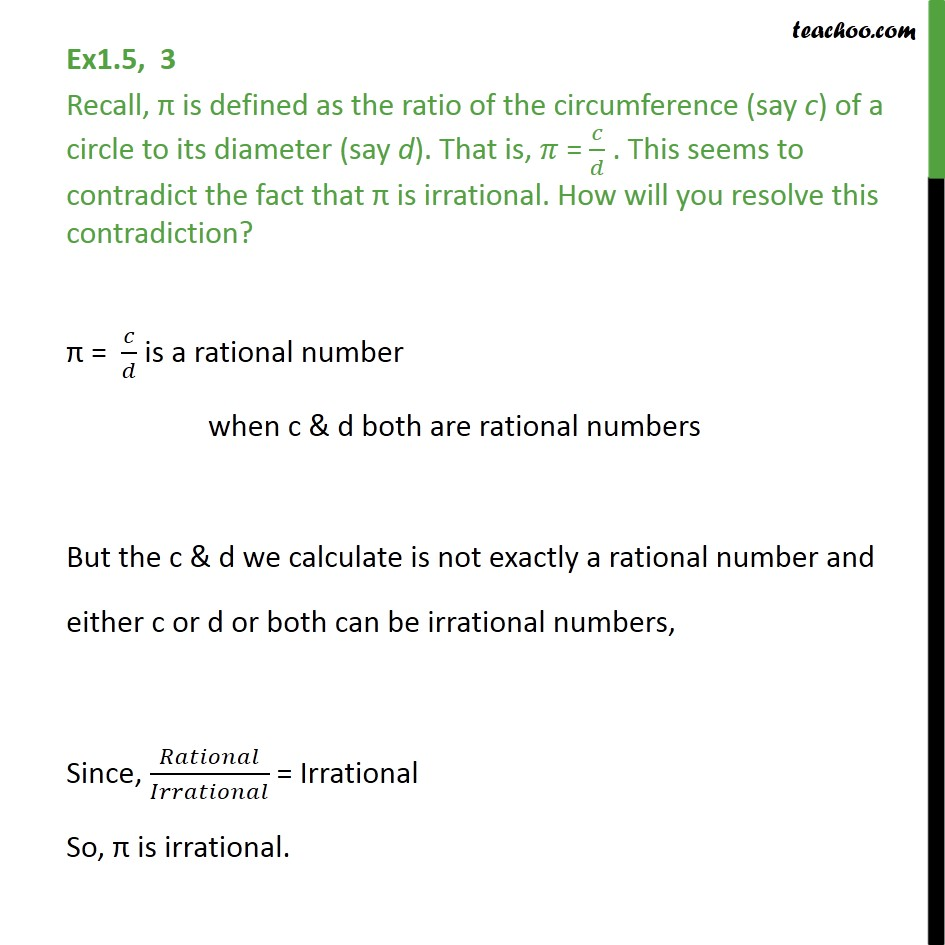Learn All Concepts of Chapter 1 Class 9 - FREE. Check - Chapter 1 Class 9 Maths1. Chapter 1 Class 9 Number Systems
2. Concept wise
3. Classifiying rational/irrational

Transcript

Ex1.5, 3 Recall, is defined as the ratio of the circumference (say c) of a circle to its diameter (say d). That is, = / . This seems to contradict the fact that is irrational. How will you resolve this contradiction? = / is a rational number when c & d both are rational numbers But the c & d we calculate is not exactly a rational number and either c or d or both can be irrational numbers, Since, / = Irrational So, is irrational.

Classifiying rational/irrational